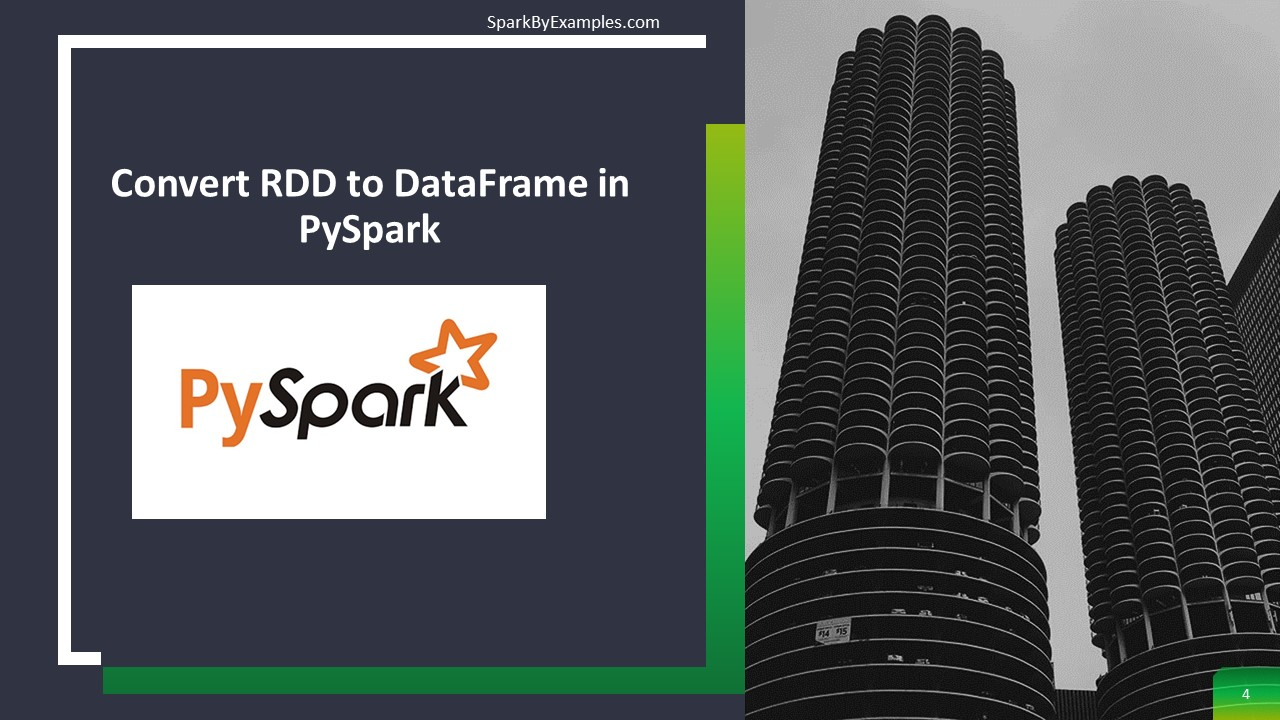# Convert PySpark RDD to DataFrame

In PySpark, `toDF()` function of the RDD is used to convert RDD to DataFrame. We would need to convert RDD to DataFrame as DataFrame provides more advantages over RDD. For instance, DataFrame is a distributed collection of data organized into named columns similar to Database tables and provides optimization and performance improvements.

## 1. Create PySpark RDD

First, let’s create an RDD by passing Python list object to `sparkContext.parallelize()` function. We would need this `rdd` object for all our examples below.

In PySpark, when you have data in a list meaning you have a collection of data in a PySpark driver memory when you create an RDD, this collection is going to be parallelized.

``````
from pyspark.sql import SparkSession
spark = SparkSession.builder.appName('SparkByExamples.com').getOrCreate()
dept = [("Finance",10),("Marketing",20),("Sales",30),("IT",40)]
rdd = spark.sparkContext.parallelize(dept)
``````

## 2. Convert PySpark RDD to DataFrame

Converting PySpark RDD to DataFrame can be done using toDF(), createDataFrame(). In this section, I will explain these two methods.

### 2.1 Using rdd.toDF() function

PySpark provides toDF() function in RDD which can be used to convert RDD into Dataframe

``````
df = rdd.toDF()
df.printSchema()
df.show(truncate=False)
``````

By default, `toDF()` function creates column names as “_1” and “_2”. This snippet yields below schema.

``````
root
|-- _1: string (nullable = true)
|-- _2: long (nullable = true)

+---------+---+
|_1       |_2 |
+---------+---+
|Finance  |10 |
|Marketing|20 |
|Sales    |30 |
|IT       |40 |
+---------+---+

``````

`toDF() `has another signature that takes arguments to define column names as shown below.

``````
deptColumns = ["dept_name","dept_id"]
df2 = rdd.toDF(deptColumns)
df2.printSchema()
df2.show(truncate=False)
``````

Outputs below schema.

``````
root
|-- dept_name: string (nullable = true)
|-- dept_id: long (nullable = true)

+---------+-------+
|dept_name|dept_id|
+---------+-------+
|Finance  |10     |
|Marketing|20     |
|Sales    |30     |
|IT       |40     |
+---------+-------+
``````

### 2.2 Using PySpark createDataFrame() function

`SparkSession `class provides `createDataFrame()` method to create DataFrame and it takes rdd object as an argument.

``````
deptDF = spark.createDataFrame(rdd, schema = deptColumns)
deptDF.printSchema()
deptDF.show(truncate=False)
``````

This yields the same output as above.

## 2.3 Using createDataFrame() with StructType schema

When you infer the schema, by default the datatype of the columns is derived from the data and set’s nullable to true for all columns. We can change this behavior by supplying schema using StructType – where we can specify a column name, data type and nullable for each field/column.

If you wanted to know more about StructType, please go through how to use StructType and StructField to define the custom schema.

``````
from pyspark.sql.types import StructType,StructField, StringType
deptSchema = StructType([
StructField('dept_name', StringType(), True),
StructField('dept_id', StringType(), True)
])

deptDF1 = spark.createDataFrame(rdd, schema = deptSchema)
deptDF1.printSchema()
deptDF1.show(truncate=False)
``````

This also yields the same output.

## 3. Complete Example

``````
import pyspark
from pyspark.sql import SparkSession

spark = SparkSession.builder.appName('SparkByExamples.com').getOrCreate()

dept = [("Finance",10),("Marketing",20),("Sales",30),("IT",40)]
rdd = spark.sparkContext.parallelize(dept)

df = rdd.toDF()
df.printSchema()
df.show(truncate=False)

deptColumns = ["dept_name","dept_id"]
df2 = rdd.toDF(deptColumns)
df2.printSchema()
df2.show(truncate=False)

deptDF = spark.createDataFrame(rdd, schema = deptColumns)
deptDF.printSchema()
deptDF.show(truncate=False)

from pyspark.sql.types import StructType,StructField, StringType
deptSchema = StructType([
StructField('dept_name', StringType(), True),
StructField('dept_id', StringType(), True)
])

deptDF1 = spark.createDataFrame(rdd, schema = deptSchema)
deptDF1.printSchema()
deptDF1.show(truncate=False)
``````